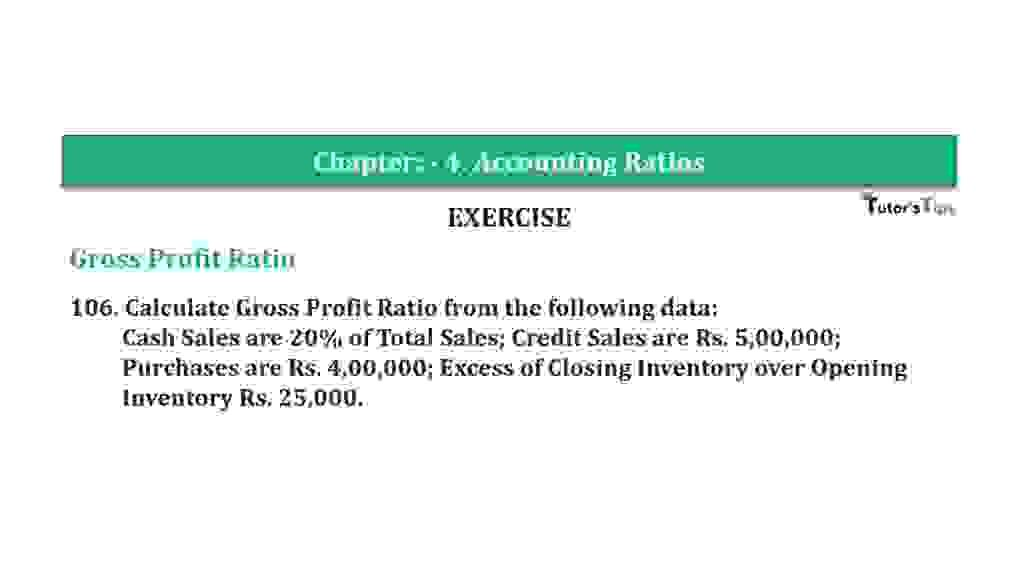# Question 106 Chapter 4 of +2-B – T.S. Grewal 12 ClassQuestion 106 Chapter 4 of +2-B

Gross Profit Ratio

106. Calculate Gross Profit Ratio from the following data:
Cash Sales are 20% of Total Sales; Credit Sales are Rs. 5,00,000;
Purchases are Rs. 4,00,000; Excess of Closing Inventory over Opening
Inventory Rs. 25,000.

### The solution of Question 106 Chapter 4 of +2-B: –

 Credit Sales = Rs. 5,00,000 Cash Sales = 20% of Total Sales Total Sales = Cash Sales + Credit Sales Total Sales = X

 X = Rs.5,00,000+ 20x 100
 X – 20x = Rs.5,00,000 100
 Rs. 4,00,000 = X + 25x 100 80x = Rs. 5,00,00,000 X = Rs. 5,00,00,000 80
 = Rs. 6,25,000 Cost of Goods Sold = Purchases – Excess of Closing Stock over Opening Stock = Rs. 4,00,000 – Rs. 25,000 = Rs. 3,75,000 Gross Profit = Total Sales – Cost of Goods Sold = Rs. 6,25,000 – Rs. 3,75,000 = Rs. 2,50,000

 Revenue from Operations = Gross Profit X 100 Net Sales
 Revenue from Operations = Rs. 2,50,000 X 100 Rs. 6,25,000 = 40%

Balance Sheet: Meaning, Format & Examples

Thanks, Please Like and share with your friends

Comment if you have any question.

Also, Check out the solved question of previous Chapters: –

### T.S. Grewal’s Double Entry Book Keeping (Vol. II: Accounting for Companies)T.S. Grewal’s Analysis of Financial Statements